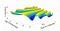# Meta-learning in finance: boosting models calibration with deep learningThe Calibration of Stochastic-Local Volatility Models — An Inverse Problem Perspective

# Machine learning for model calibration

`def dense_bn_block(inn, size):    x = Dense(size, activation='linear')(inn)    x = BatchNormalization()(x)    x = Activation('relu')(x)    return xdef residual_block(inn, size):    x = dense_bn_block(inn, size)    x = add([inn, x])    return xinputs = Input(shape=(295, ))x = GaussianNoise(0.05)(inputs)x = BatchNormalization()(x)for i in range(1, 5):    x = residual_block(x, 64)    x = Dropout(0.25)(x)predictions = Dense(1, activation='linear')(x)model = Model(inputs=inputs, outputs=predictions)`